Rank Correlation - Correlation & Regression, Business Mathematics & Statistics

# Rank Correlation - Correlation & Regression, Business Mathematics & Statistics - Business Mathematics and Statistics - B Com

Rank Correlation Rank method for the computation of the coefficient of correlation is based on the rank or the order & not the magnitude of the variable. Accordingly it is more suitable when the variables can be arranged for e.g. in case of intelligence or beauty or any other qualitative phenomenon. The ranks may range from 1 to n.
Edward spearman has provided the following formula —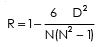Where N = Number of pairs of variable X & Y
D = Rank difference

Example 4 : From the data given belows calculate the rank correlation between x & Y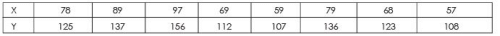Solution :
Table :
Computation of Rank Correlation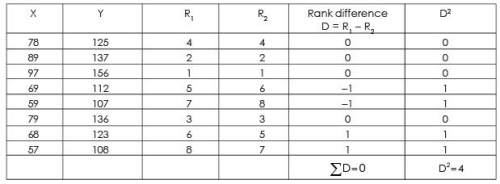Rank correlation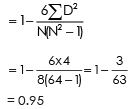This shows there is very high positive correlation between X & Y.

Example 5 : Calculate Rank Correlation from the following data.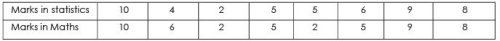Solution : Table : Calculation of Rank correlation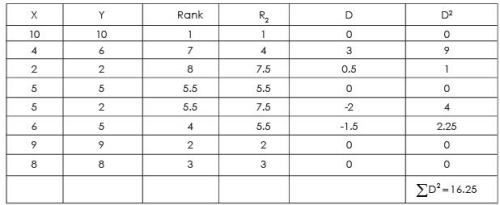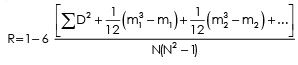Here m, m2 ... denote the number of times ranks are tied in both the variables, the subscripts & denote the first tie, second tie,...., in both the variables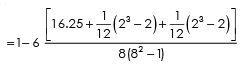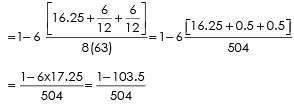= 1 – 0.205
= 0.795

Example 6 : Find the coefficient of correlation between price and sales from the following data :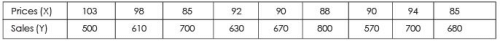Solution : Let the value of assumed mean for X(AX) be 90
Let the value of assumed mean for Y(Ay) be 700
Table : Calculation of correlation coefficient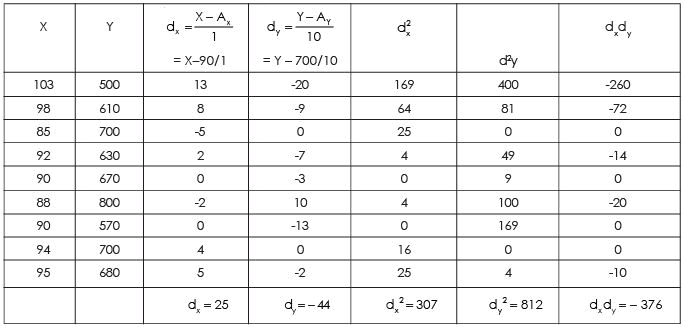Note : As r is a pure number, change of scale does not affect its value. Hence the values are divided by 10 in column 4 to make the calculations simple. The following formula can be applied to all the problems.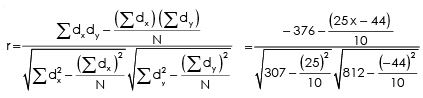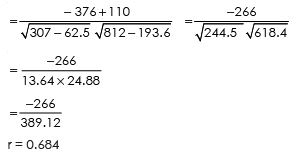Steps.
(i) find out the direction of change of X variable, i.e., as compared with the first value, whether the second value is increasing or decreasing or is constant. If it is increasing put (+) sign; if it is decreasing put (-) sign (minus) and if it is constant put zero. Similarly, as compared to second  value find out whether the third value is increasing, decreasing or constant. Repeat the same process for other values. Denote this column by Dx.
(ii) In the same manner as discussed above find out the direction of change of Y variable and denote this column by Dy.
(iii) Multiply Dx with Dy, and determine the value of c, i.e., the number of positive signs.
(iv) Apply the above formula,  i.e.,
rc = +√+ (2C-n)/n
Note. The significance of + signs, both (inside the under root  and outside the under root) is that we cannot take the under root of minus sign. Therefore, if 2C-n is negative, this negative n value of multiplied with the minus sign inside would make it positive and we can take the under root. But the ultimate result would be negative. If 2C-n  is positive then, of course, we get a positive n value of the coefficient of correlation.
For more help in Concurrent Deviation Method please click the button below to submit your homework assignment.

The document Rank Correlation - Correlation & Regression, Business Mathematics & Statistics | Business Mathematics and Statistics - B Com is a part of the B Com Course Business Mathematics and Statistics.
All you need of B Com at this link: B Com

115 videos|142 docs

## FAQs on Rank Correlation - Correlation & Regression, Business Mathematics & Statistics - Business Mathematics and Statistics - B Com

 1. What is rank correlation?Ans. Rank correlation is a statistical measure used to determine the strength and direction of the relationship between two variables by comparing their ranks. It does not require the variables to be measured on a specific scale, making it suitable for non-parametric data analysis.
 2. How is rank correlation different from other types of correlation?Ans. Rank correlation differs from other types of correlation, such as Pearson correlation, as it focuses on the ranks of the data rather than the actual values. This makes it a useful tool for analyzing data that may not have a linear relationship or when the data does not meet the assumptions of parametric tests.
 3. How is rank correlation calculated?Ans. Rank correlation is typically calculated using statistical methods such as Spearman's rank correlation coefficient or Kendall's rank correlation coefficient. These methods assign ranks to the data, and then calculate the correlation based on the difference between the ranks of the two variables.
 4. What does a positive rank correlation coefficient indicate?Ans. A positive rank correlation coefficient indicates a positive relationship between the two variables being analyzed. This means that as the rank of one variable increases, the rank of the other variable also tends to increase. However, it does not imply causation, and the strength of the relationship is determined by the magnitude of the coefficient.
 5. Why is rank correlation useful in business mathematics and statistics?Ans. Rank correlation is useful in business mathematics and statistics as it allows for the analysis of data that may not conform to the assumptions of parametric tests. It can be used to determine if there is a relationship between variables, even if the relationship is not linear. This makes it a valuable tool in analyzing data and making informed business decisions.

115 videos|142 docsExplore Courses for B Com examSignup to see your scores go up within 7 days! Learn & Practice with 1000+ FREE Notes, Videos & Tests.
10M+ students study on EduRev
Track your progress, build streaks, highlight & save important lessons and more!
Related Searches

,

,

,

,

,

,

,

,

,

,

,

,

,

,

,

,

,

,

,

,

,

,

,

,

;